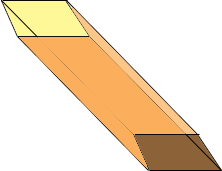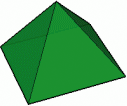SEARCH HOMEMath Central Quandaries & QueriesQuestion from Sheyna, a student: What are the differences between a square prism and a pyramid? - Sheyna ( Please answer I really need this ) ThanksSheyna,

A square prism has a square base, a congruent square top and the sides are parallelograms. For example a pizza box is a square prism. The sides are special parallelograms, they are rectangles (and very short). Your refrigerator is approximately a square prism if the top and bottom are squares. Here again the sides are rectangles. The prism might be "tilted" (as long as the base and top are congruent squares) so that the sides are parallelograms but not rectangles.A square pyramid has a square base and its four sides are triangles, all of which have a common vertex.PennyMath Central is supported by the University of Regina and The Pacific Institute for the Mathematical Sciences.